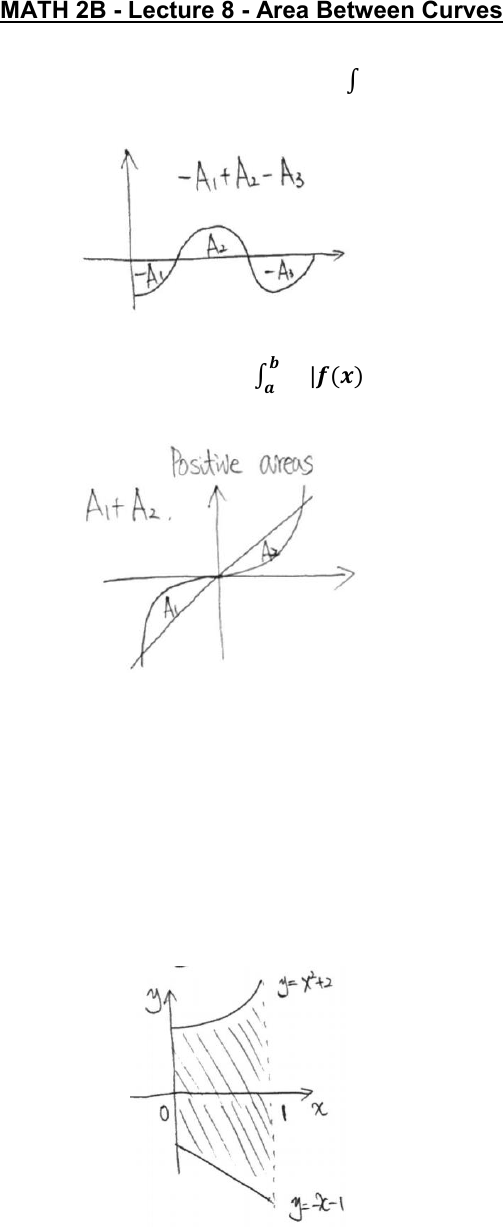Class Notes (1,100,000)
US (460,000)
UC-Irvine (10,000)
MATH (1,000)
MATH 2B (600)
Lecture 8

# MATH 2B Lecture 8: Area Between CurvesPremium

Department
Mathematics
Course Code
MATH 2B
Professor
ERJAEE, G.
Lecture
8

This preview shows page 1. to view the full 4 pages of the document.01/23/2019
MATH 2B - Lecture 8 - Area Between Curves
Definite integral of a function: 𝑏
𝑎𝑓(𝑥)𝑑𝑥
Net Area
Area between curves: 𝒃
𝒂|𝒇(𝒙)𝒈(𝒙)|𝒅𝒙
Positive areas
To make sure all the areas are positive, we need to plot the two curves and decide
which function is on the top, which is at the bottom.
Case I: One function is always on the top on the whole interval.
Example:
Find the area between y=x²+2 and y=-x-1, on the interval [0,1].